Basics of Geometry
edHelper subscribers - Create a new printable

Number of Keys
 Select the number of different printables: 1 key 2 keys 3 keys 4 keys 5 keys

Number of Pages (for each key)
 Select the number of pages: 1 page 2 pages 3 pages 4 pages

Include an answer key (answer keys will be at the end of the printable)
High School Geometry
High School Geometry
Math

 Name _____________________________Date ___________________ (Key 1 - Answer ID # 1045921)
1.   The diagonals of a parallelogram ___________.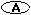have the same length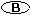bisect each other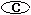are congruent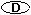are perpendicular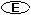None of the above
2.   A ___________ is a quadrilateral having two pairs of parallel sides.parallelogramheptagontrapezoidpolygonNone of the above
3.   In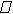LOCG the sum of the measures of angle L and angle C is 288. If L = (157 + x), what is the value of x?
4.   In parallogramOHFG, the measure of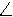F exceeds the measure ofH by 58. What is the measure ofF?
5.   Consecutive pairs of angles of a parallelogram are ___________.complementaryparallelsupplementaryperpendicularcongruent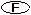None of the above
6.   A quadrilateral with two pairs of parallel sides and four congruent sides is a ___________.trapezoidsquarerhombusrectangleNone of the above
7.   In parallogramESHL, a diagonal SL is drawn. The measure ofSHL = 112and the measure ofSLH = 50. What is the measure ofSLE?
8.   The perimeter ofGTFP is 192 more than the length of side GT and 159 more than the length of side TF. What is the length of side FP?
9.   Two diagonals, which meet at G are drawn inKLFB. The length of KL is 7. The sum of the total length of the diagonals KF and LB is 42. The length of LG is 12.5. What is the length of KF?
10.   InHKOS a line KQ is drawn fromK perpendicular to HS. If the measure ofO = 49then what is the measure ofHKQ?

Basics of Geometry
edHelper subscribers - Create a new printable

Number of Keys
 Select the number of different printables: 1 key 2 keys 3 keys 4 keys 5 keys

Number of Pages (for each key)
 Select the number of pages: 1 page 2 pages 3 pages 4 pages